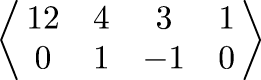Next Continued Fraction Arithmetic 39

# Arithmetic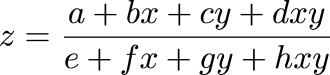• To compute x + y, start with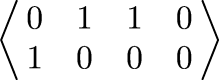• To compute x - y, start with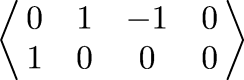• To compute x · y, start with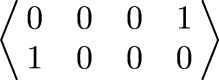• To compute x ÷ y, start with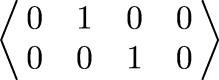• And interesting shortcuts are available:

• To compute (x + 3)(y + 4) / (x - y), do not do a multiplication, a subtraction, and a division